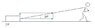# Man pulling a cube on a rough surface

• Karol

## Homework Statement

A man of mass 70[kg] pules a box at an angle 100. the static coefficient of friction between his legs and the floor is 0.6 and the kinetic coefficient between the box and the floor is 0.3
What's the maximum's box's mass

Friction: f=μN

## The Attempt at a Solution

The horizontal force the man can apply:
$$70\cdot 9.81\cdot 0.6=412.02=F\cdot \cos 10^0\rightarrow F=418.38$$
The vertical component of F raises the box: ##F\cdot \sin 10^0=418.02\cdot\sin 10^0=72.65##
the force needed to pull the box:
$$(mg-F\cdot \sin 10^0)0.3=412.02\rightarrow (mg-72.65)0.3=412.02\rightarrow m=147.4[kg]$$
It should be 164.85

#### Attachments

•Snap1.jpg
4.9 KB · Views: 353
The vertical component of F does more than just that !

The vertical component also presses down on the man:
$$\left\{ \begin{array}{l} (mg-F\cdot \sin10^0)0.3=(70\cdot g+F\cdot \sin10^0)0.6 \\ F\cdot \cos10^0=(mg-F\cdot \sin10^0)0.3 \end{array} \right.$$
$$\rightarrow F=467.88,\ m=164.87\ \surd$$